## Example Questions

1 3 Next →

### Example Question #40 : Complex Numbers

Raise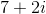to the fourth power.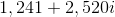None of these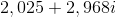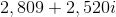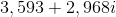Explanation:

By the Power of a Power Rule, the fourth power of any number is equal to the square of the square of that number: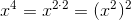Therefore, one way to raiseto the fourth power is to square it, then to square the result.

Using the binomial square pattern to square: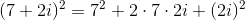Applying the Power of a Product Property: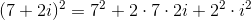Since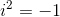by definition: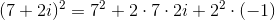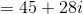Square this using the same steps: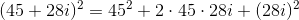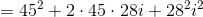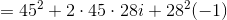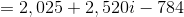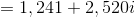### Example Question #51 : Squaring / Square Roots / Radicals

Raise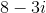to the fourth power.

None of these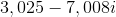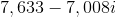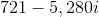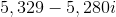Explanation:

The easiest way to find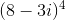is to note that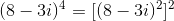.

Therefore, we can find the fourth power ofby squaring, then squaring the result.

Using the binomial square pattern to square: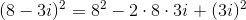Applying the Power of a Product Property: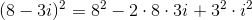Sinceby definition: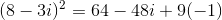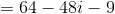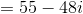Square this using the same steps: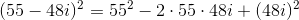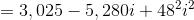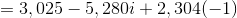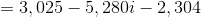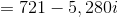Therefore,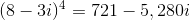### Example Question #41 : Complex Numbers

Raise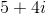to the third power.

None of these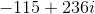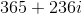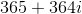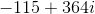Explanation:

To raise any expression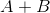to the third power, use the pattern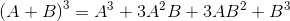Setting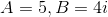: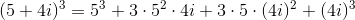Taking advantage of the Power of a Product Rule: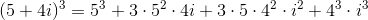Sinceand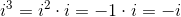: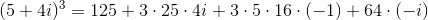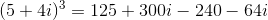Collecting real and imaginary terms: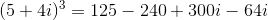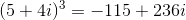### Example Question #51 : Squaring / Square Roots / Radicals

Evaluate: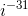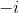The expression is undefinedExplanation: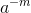is defined to be equal to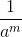for any real or imaginaryand for any real; therefore,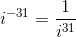To evaluate a positive power of, divide the power by 4 and note the remainder: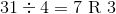Therefore,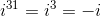Substituting,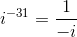Rationalizing the denominator by multiplying both numerator and denominator by: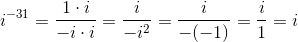1 3 Next →

### All SAT Math Resources